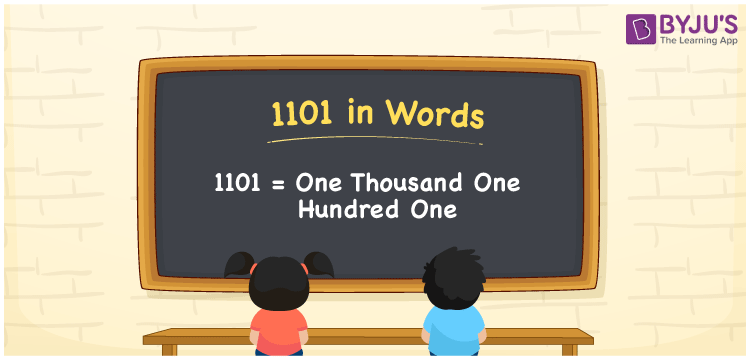# 1101 in Words

We can write 1101 in words as One thousand one hundred one. We can quickly transform the number 1101 into words with the help of a place value chart. If you paid an Rs. 1101 bill in a cafeteria, you can say, “I spent One thousand one hundred one rupees bill at the cafeteria”. In this article, you will learn how to write the cardinal number 1101 in word form in an easily understandable method.

 1101 in words One thousand one hundred one One thousand one hundred one in Numerical Form 1101

## 1101 in English Words

We generally express numbers in words using the English alphabet. Thus, we can read 1101 in English as “One thousand one hundred one”.## How to Write 1101 in Words?

1101 is a four-digit number. So, we can convert the number 1101 into word form using a four-column place value chart as shown below.

 Thousands Hundreds Tens Ones 1 1 0 1

Let us write the expanded form of 1101 from the above table.

1 × Thousand + 1 × Hundred + 0 × Ten + 1 × One

= 1 × 1000 + 1 × 100 + 0 × 10 + 1 × 1

= 1000 + 100 + 1

= One thousand + One hundred + One

= One thousand one hundred one

Hence, 1101 in words = One thousand one hundred one.

As we know, 1101 is a natural number that precedes 1102 and succeeds 1100.

1101 in words – One thousand one hundred one

Is 1101 an even number? – No

Is 1101 an odd number? – Yes

Is 1101 a perfect square number? – No

Is 1101 a perfect cube number? – No

Is 1101 a prime number? – No

Is 1101 a composite number? – Yes

## Frequently Asked Questions on 1101 in Words

Q1

### How do you write 1101 in words?

Generally, we can write 1101 in words as One thousand one hundred one.
Q2

### What is the number name for 1101?

One thousand one hundred one is the number name for 1101.
Q3

### How to write Rs. 1101 in words on a cheque?

On a cheque, we must write 1101 in words as “One thousand one hundred one rupees only”.
Test your Knowledge on 1101 in Words